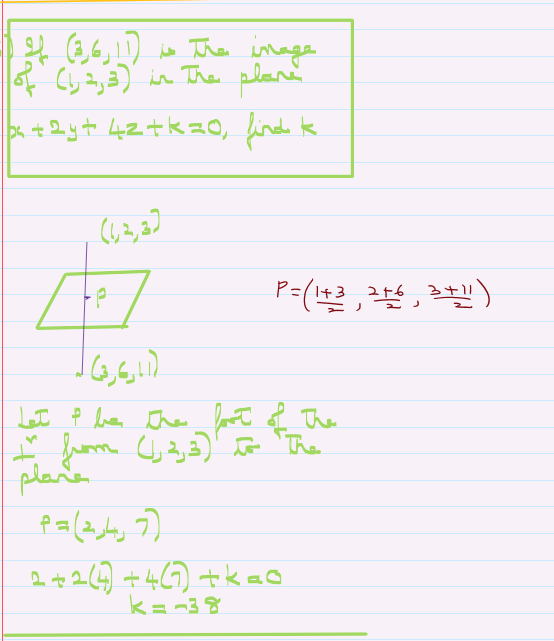Search

# Image of a point in a plane-3 Dimensional Geometry-Math-Class 12

A simple problem in 3 dimensional geometry where you learn that image of a point in a plane is as simple as using the midpoint formula. A great tool for math students of class 12.

For individual help, you can message me on suman@mathews.co.in